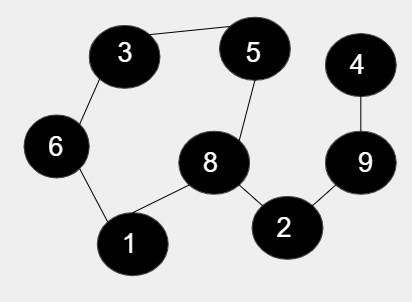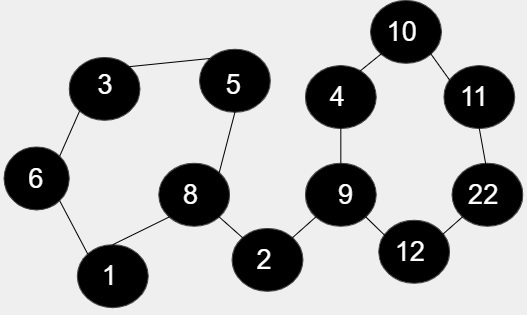# Product of lengths of all cycles in an undirected graph in C++

We are given with the undirected as well as unweighted graph as an input and the task is to find the product of the cycles that are formed in the given and display the result.

## Example

InputIn the given figure, there are 8 nodes and out of that 5 nodes are forming cycle including 1, 6, 3, 5, 8 and rest of the node are not included in the cycle. So, the length of the cycle is 5 as it includes 5 node therefore product is 5In the given figure, there are 12 nodes and out of that 11(5 +6) nodes are forming cycle including 1, 6, 3, 5, 8 and 9, 4, 10, 11, 22, 12 and the rest of the node 2 is not included in the cycle. So, the length of the cycle is 5 * 6 = 30

Approach used in the below program is as follows

• Input the nodes for forming the cycle
• Create the DFS function and call it to traverse the vertex by coloring it
• Either the node are marked completely visited or partially visited
• Completely visited node need not be visit again and hence need not to store it whereas partially visited nodes need to be stored because they are visited again
• Print the result

## Algorithm

Start
Step 1-> declare function to traverse the graph using DFS approach
void DFS(int i, int j, int color[], int highlight[], int parent[], int& number)
IF color[i] = 2
Return
End
IF color[i] = 1
Set number++
Declare and set int temp = j
Set highlight[temp] = number
Loop While temp != i
Set temp = parent[temp]
Set highlight[temp] = number
End
Return
End
Set parent[i] = j
Set color[i] = 1
For int k : graph[i]
IF k = parent[i]
Continue
End
Call DFS(k, i, color, highlight, parent, number)
End
Set color[i] = 2
Step 2-> declare function to find product of nodes in cycle
int product(int edge, int highlight[], int& number)
call unordered_map<int, int> mp
Loop For i = 1 and i <= edge and i++
IF (highlight[i] != 0)
Set mp[highlight[i]]++
End
End
Declare and set int temp = 1
Loop For i = 1 and i <= number and i++
Set temp = temp * mp[i]
End
IF number = 0
Set temp = 0
End
return temp
Step 3-> In main()
Call function as insert(1, 2) to insert a node
Declare int color[size], parent[size]
Declare int highlight[size]
Declare and set int number = 0
Declare and set int edge = 10
Call DFS(1, 0, color, highlight, parent, number)
Call print function as product(edge, highlight, number)
Stop

## Example

Live Demo

#include <bits/stdc++.h>
using namespace std;
const int size = 100000;
vector<int> graph[size];
//function to traverse the graph using DFS approach
void DFS(int i, int j, int color[], int highlight[], int parent[], int& number) {
// for travered node
if (color[i] == 2) {
return;
}
//not completely visited
if (color[i] == 1) {
number++;
int temp = j;
highlight[temp] = number;
//for backtracking the vertex
while (temp != i) {
temp = parent[temp];
highlight[temp] = number;
}
return;
}
parent[i] = j;
color[i] = 1;
for (int k : graph[i]) {
if (k == parent[i]) {
continue;
}
DFS(k, i, color, highlight, parent, number);
}
color[i] = 2;
}
// function for inserting edges to graph
void insert(int u, int v) {
graph[u].push_back(v);
graph[v].push_back(u);
}
// Find product of nodes in cycle
int product(int edge, int highlight[], int& number) {
unordered_map<int, int> mp;
for (int i = 1; i <= edge; i++) {
if (highlight[i] != 0)
mp[highlight[i]]++;
}
int temp = 1;
for (int i = 1; i <= number; i++) {
temp = temp * mp[i];
}
if (number == 0)
temp = 0;
return temp;
}
int main() {
//for inserting a node in the graph
insert(1, 2);
insert(2, 3);
insert(3, 4);
insert(4, 6);
insert(4, 7);
insert(5, 6);
insert(3, 5);
insert(7, 8);
insert(6, 10);
insert(5, 9);
insert(10, 11);
int color[size], parent[size];
int highlight[size];
int number = 0;
int edge = 10;
DFS(1, 0, color, highlight, parent, number);
// function to print the cycles
cout<<"product of all the nodes in the cycle is :"<< product(edge, highlight, number);
return 0;
}

## Output

Product of all the nodes in the cycle is :4